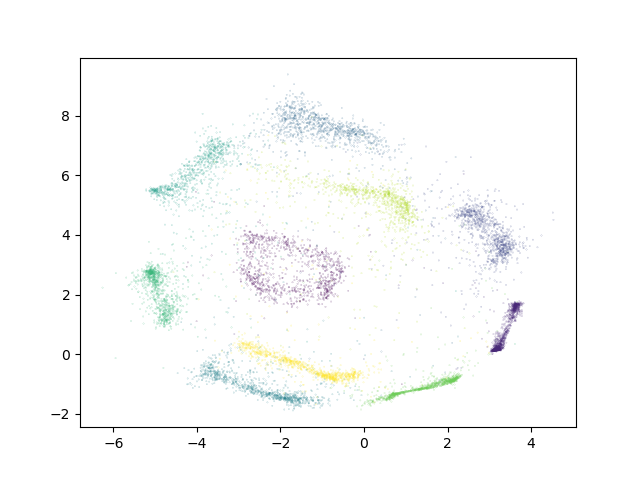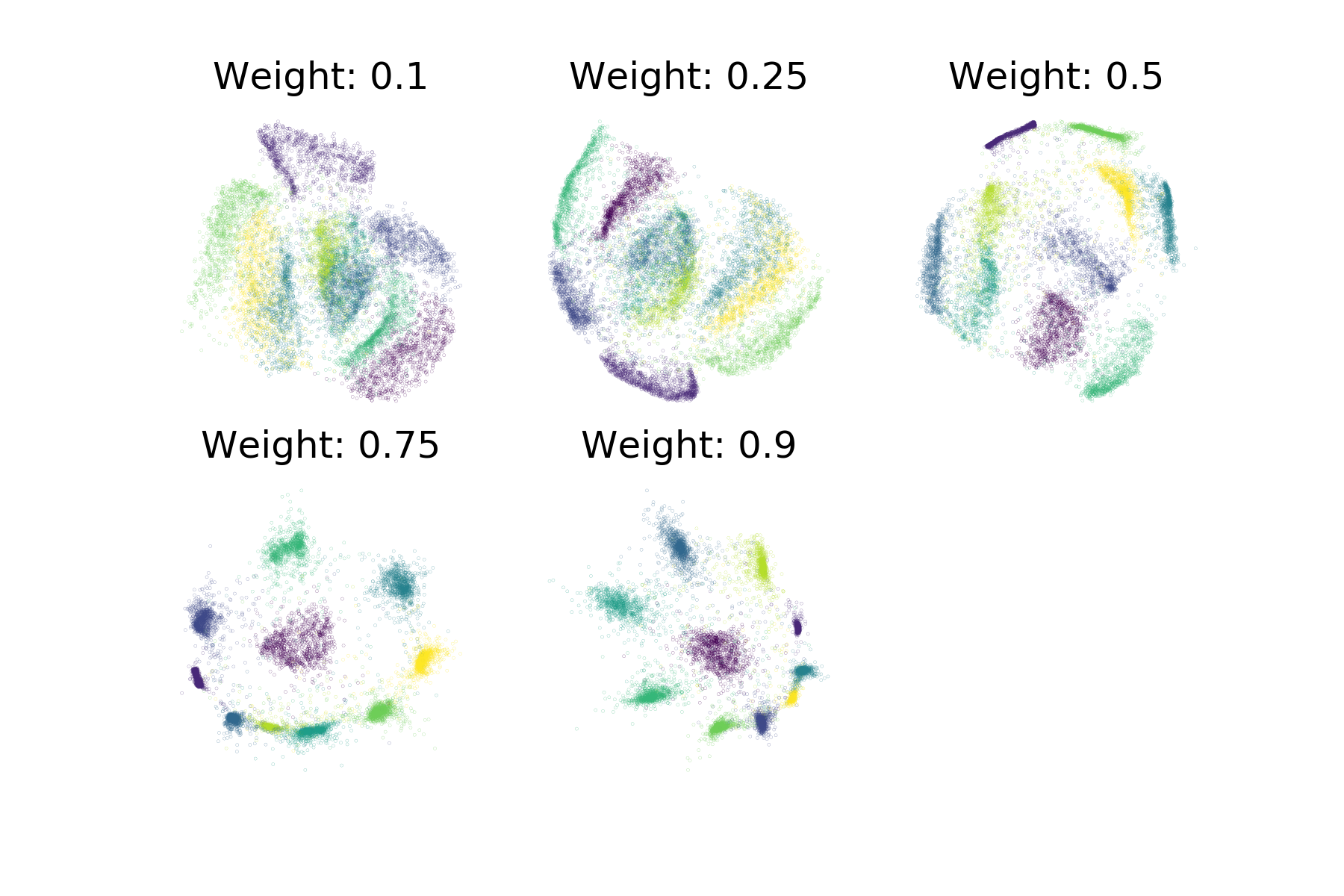# Semi-supervised Dimensionality Reduction¶

Sometimes only part of a dataset has ground-truth labels available. In such a scenario, `ivis` is still able to make use of existing label information in conjunction with the inputs to do dimensionality reduction when in semi-supervised mode. When in semi-supervised mode, `ivis` will use labels when available as well as the unsupervised triplet loss. However, when label information is not available, only the unsupervised loss will be used. By training in semi-supervised mode, we can make full use of the data available, even if it is only partially labeled.

In order to use semi-supervised learning, mark missing labeled points as -1 in the Y vector provided to `ivis` when calling fit or fit_transform. Currently, only sparse_categorical_crossentropy loss works with semi-supervised inputs.

## Semi-supervised Classification¶

To train `ivis` in semi-supervised mode using the default softmax classification loss, simply provide the labels to the fit method’s `Y` parameter. These labels should be a list of 0-indexed integers with each integer corresponding to a class. Missing labels should be denoted with -1.

In the example below, we will mask 50% of the available labels for the MNIST dataset.

```import numpy as np
from tensorflow.keras.datasets import mnist
from ivis import Ivis

(X_train, Y_train), (X_test, Y_test)  = mnist.load_data()

# Rescale to [0,1]
X_train = X_train / 255.
X_test = X_test / 255.

# Flatten images to 1D vectors
X_train = np.reshape(X_train, (len(X_train), 28 * 28))
X_test = np.reshape(X_test, (len(X_test), 28 * 28))

mask = np.random.choice(range(len(Y_train)), size=len(Y_train) // 2, replace=False)
Y_train_masked = np.array(Y_train, dtype=np.int8) # Can't use uint to represent negative numbers

model = Ivis(n_epochs_without_progress=5)
```

Experimental data has shown that `ivis` converges to a solution faster in supervised mode. Therefore, our suggestion is to lower the value of the `n_epochs_without_progress` parameter from the default to around 5. Here are the resulting embeddings on the testing set:## Supervision Weight¶

As in supervised mode, it is still possible to control the relative importance `ivis` places on the labels when training in supervised mode with the `supervision_weight` parameter. This variable should be a float between 0.0 to 1.0, with higher values resulting in supervision affecting the training process more, and smaller values resulting in it impacting the training less. By default, the parameter is set to 0.5. Increasing it will result in more cleanly separated classes.

```weight = 0.8
model = Ivis(n_epochs_without_progress=5,
supervision_weight=weight)
model.fit(X_train, Y_train)
```

As an illustration of the impact the `supervision_weight` has on the resulting embeddings, see the following plot of supervised `ivis` applied to MNIST with different weight values:In semi-supervised mode, the supervision weight may need to be higher to have the same effect on the resulting embeddings as in supervised mode, depending on the dataset. This is because when unlabeled points are encountered, unsupervised loss will still have an impact, while the supervised loss will not apply. The more of the dataset is unlabeled, the higher the supervision weight should be to have an impact on the embeddings.